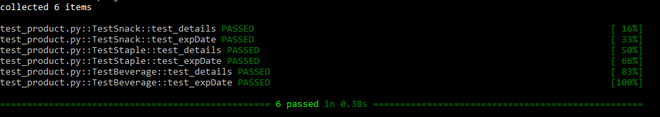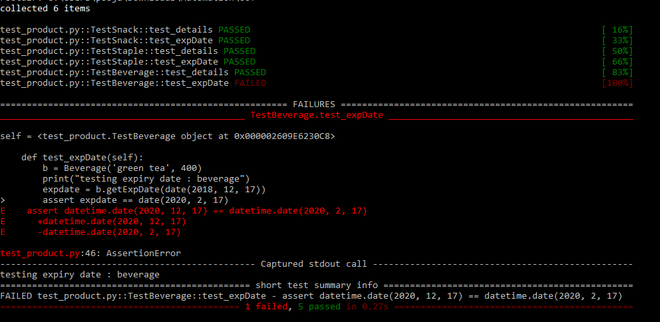# Object oriented testing in Python

Prerequisite: Object-Oriented Testing

Automated Object-Oriented Testing can be performed in Python using Pytest testing tool. In this article, we perform object-oriented testing by executing test cases for classes. We write a program that has one parent class called Product and three child classes – Snack, Beverage, and Staples. We implement all the classes and save them in a file called product.py. The classes have the following functions:

• Snack – printDetails() and getExpDate()
• Beverage – printDetails() and getExpDate()
• Staples – printDetails() and getExpDate()

It is important to note that getExpDate() is an overridden function in this case.

Code in product.py file:

## Python3

 `# importing the modules ` `from` `datetime ``import` `date ` `from` `dateutil.relativedelta ``import` `relativedelta ` ` `  `# base class ` ` `  ` `  `class` `Product: ` ` `  `    ``name ``=` `"" ` ` `  `    ``# printing the class in the constructor ` `    ``def` `__init__(``self``): ` `        ``print``(``"super class Product"``) ` ` `  `# getExpDate() returns the expiry date of product ` `# since every product has different expiry date ` `# therefore this method is overridden by child classes ` `    ``def` `getExpDate(): ` ` `  `        ``# gives exp date ` `        ``print``(``"Expiry date"``) ` `        ``pass` ` `  ` `  `# derived class 1 ` `class` `Snack(Product): ` ` `  `    ``# months ` `    ``shelfLife ``=` `6` `    ``price ``=` `0` ` `  `    ``# constructor - initializing variables ` `    ``def` `__init__(``self``, name, price): ` `        ``self``.name ``=` `name ` `        ``self``.price ``=` `price ` ` `  `    ``# prints the Snack product details ` `    ``def` `printDetails(``self``): ` `        ``print``(``"name : "` `+` `self``.name) ` `        ``print``(``"price : "` `+` `str``(``self``.price)) ` `        ``print``(``"shelf life : "` `+` `str``(``self``.shelfLife) ``+` `" months"``) ` ` `  `    ``# calculates the expiry date using relativedelta library and returns ` `    ``def` `getExpDate(``self``, pkdDate): ` `        ``expDate ``=` `pkdDate ``+` `relativedelta(months``=``self``.shelfLife) ` `        ``return` `expDate ` ` `  `# derived class 2 ` `class` `Beverage(Product): ` ` `  `    ``# 2 years ` `    ``shelfLife ``=` `2` `    ``price ``=` `0` ` `  `    ``# constructor - initializing variables ` `    ``def` `__init__(``self``, name, price): ` `        ``self``.name ``=` `name ` `        ``self``.price ``=` `price ` ` `  `    ``# prints the Beverage product details ` `    ``def` `printDetails(``self``): ` `        ``print``(``"name : "` `+` `self``.name) ` `        ``print``(``"price : "` `+` `str``(``self``.price)) ` `        ``print``(``"shelf life : "` `+` `str``(``self``.shelfLife) ``+` `" years"``) ` ` `  `    ``# calculates the expiry date using relativedelta  ` `    ``# library and returns ` `    ``def` `getExpDate(``self``, pkdDate): ` `        ``expDate ``=` `pkdDate ``+` `relativedelta(years``=``self``.shelfLife) ` `        ``return` `expDate ` ` `  ` `  `# derived class 3 ` `class` `Staples(Product): ` ` `  `    ``# 1 year ` `    ``shelfLife ``=` `1` `    ``price ``=` `0` ` `  `    ``# constructor - initializing variables ` `    ``def` `__init__(``self``, name, price): ` `        ``self``.name ``=` `name ` `        ``self``.price ``=` `price ` ` `  `    ``# prints the Staples product details ` `    ``def` `printDetails(``self``): ` `        ``print``(``"name : "` `+` `self``.name) ` `        ``print``(``"price : "` `+` `str``(``self``.price)) ` `        ``print``(``"shelf life : "` `+` `str``(``self``.shelfLife) ``+` `" year"``) ` ` `  `    ``# calculates the expiry date using relativedelta ` `    ``# library and returns ` `    ``def` `getExpDate(``self``, pkdDate): ` `        ``expDate ``=` `pkdDate ``+` `relativedelta(years``=``self``.shelfLife) ` `        ``return` `expDate ` ` `  ` `  `def` `main(): ` `    ``s ``=` `Snack(``'cookies'``, ``60``) ` `    ``s.printDetails() ` `    ``print``(s.name ``+` `" will expire on "` `+` `          ``str``(s.getExpDate(date(``2019``, ``10``, ``3``))) ``+` `"months"``) ` `    ``# yyyy-mm-dd ` ` `  `    ``p ``=` `Product() ` ` `  `    ``st ``=` `Staples(``'rice'``, ``300``) ` `    ``st.printDetails() ` `    ``print``(st.name ``+` `" will expire on "` `+` `str``(st.getExpDate(date(``2020``, ``1``, ``23``)))) ` ` `  `    ``b ``=` `Beverage(``'coffee'``, ``250``) ` `    ``b.printDetails() ` `    ``print``(b.name ``+` `" will expire on "` `+` `str``(b.getExpDate(date(``2018``, ``12``, ``17``)))) ` ` `  `    ``print``(``"done till here"``) ` ` `  ` `  `if` `__name__ ``=``=` `'__main__'``: ` `    ``main() `

Output:

```name : cookies
price : 60
shelf life : 6 months
super class Product
name : rice
price : 300
shelf life : 1 year
rice will expire on 2021-01-23
name : coffee
price : 250
shelf life : 2 years
coffee will expire on 2020-12-17
done till here
```

To perform object-oriented testing, we write test cases for each of the classes. The following things should be kept in mind while writing these test cases:

• Create a separate test class for testing the functions of each class, for e.g. TestSnack, TestStaple, TestBeverage
• Write a test case for each function of a class
• Use assert keyword to add assertions in the test cases. The assert statement will return True if the test case passes or will return False if the test case fails

We have written test cases for each function present in all classes of product.py file except the Product class (parent class). This is because the Product class has just one function and that too has been overridden by the child classes, so writing a test case would make no difference. Therefore, we write 6 test cases in total where two test cases are written for each of the child classes.

Code in test_product.py file:

## Python3

 `# importing the modules ` `import` `pytest ` `from` `product ``import` `Snack, Staples, Beverage ` `from` `datetime ``import` `date ` ` `  ` `  `class` `TestSnack: ` ` `  `      ``# test case for print details of Snack ` `    ``def` `test_details(``self``): ` `        ``s ``=` `Snack(``'chips'` `, ``50``) ` `        ``print``(``"testing details : snack"``) ` `        ``assert` `(``'chips'` `, ``50``, ``6``)  ``=``=` `(s.name, s.price, s.shelfLife) ` ` `  `    ``# test case for calculating exiry date of Snack ` `    ``def` `test_expDate(``self``): ` `        ``s ``=` `Snack(``'wafers'``, ``40``) ` `        ``print``(``"testing expiry date : snack"``) ` `        ``expdate ``=` `s.getExpDate(date(``2019``, ``10``, ``3``)) ` `        ``assert` `expdate ``=``=` `date(``2020``, ``4``, ``3``) ` ` `  ` `  `class` `TestStaple: ` ` `  `      ``# test case for print details of Staples ` `    ``def` `test_details(``self``): ` `        ``st ``=` `Staples(``'rice'` `, ``300``) ` `        ``print``(``"testing details : staples"``) ` `        ``assert` `(``'rice'` `, ``300``, ``1``)  ``=``=` `(st.name, st.price, st.shelfLife) ` ` `  `    ``# test case for calculating exiry date of Staples ` `    ``def` `test_expDate(``self``): ` `        ``st ``=` `Staples(``'wheat flour'``, ``400``) ` `        ``print``(``"testing expiry date : staples"``) ` `        ``expdate ``=` `st.getExpDate(date(``2020``, ``1``, ``23``)) ` `        ``assert` `expdate ``=``=` `date(``2021``, ``1``, ``23``) ` ` `  ` `  ` `  `class` `TestBeverage: ` ` `  `      ``# test case for print details of Beverage ` `    ``def` `test_details(``self``): ` `        ``b ``=` `Beverage(``'coffee'` `, ``250``) ` `        ``print``(``"testing details : beverage"``) ` `        ``assert` `(``'coffee'` `, ``250``, ``2``)  ``=``=` `(b.name, b.price, b.shelfLife) ` ` `  `    ``# test case for calculating exiry date of Beverage ` `    ``def` `test_expDate(``self``): ` `        ``b ``=` `Beverage(``'green tea'``, ``400``) ` `        ``print``(``"testing expiry date : beverage"``) ` `        ``expdate ``=` `b.getExpDate(date(``2018``, ``12``, ``17``)) ` `        ``assert` `expdate ``=``=` `date(``2020``, ``12``, ``17``)`

Note: The function name and the test file name should always start with the word ‘test‘.

To execute the above test cases, create two separate files, product.py and test_product.py in a single folder. To execute write the following command:

```pytest
```

OR

```pytest -v
```

pytest -v  shows the verbose output.

The output is shown below:If we change the value in one of the assertion statements of a test case, it will lead the test case to fail. Refer to the output shown below:In the output shown above, test_expDate() test case in class TestBeverage failed as the assertion statement resulted in a False expression.

Attention geek! Strengthen your foundations with the Python Programming Foundation Course and learn the basics.

To begin with, your interview preparations Enhance your Data Structures concepts with the Python DS Course.

My Personal Notes arrow_drop_upCheck out this Author's contributed articles.

If you like GeeksforGeeks and would like to contribute, you can also write an article using contribute.geeksforgeeks.org or mail your article to contribute@geeksforgeeks.org. See your article appearing on the GeeksforGeeks main page and help other Geeks.

Please Improve this article if you find anything incorrect by clicking on the "Improve Article" button below.

Article Tags :

Be the First to upvote.

Please write to us at contribute@geeksforgeeks.org to report any issue with the above content.